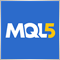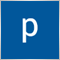# Scripts: LotLoss172265

Simple risk management tool.

Author: Mokara11

hello Mokara thanks for this script... i'm trying to add some variables in your script to consider the risk as percentage. but the result is not showing up in metatrader after running my edited script.

any opinion from this community is much appreciated.

```#property copyright           "Mokara"
#property description         "LotLoss"
#property version             "1.0"
#property script_show_inputs

input int vLoss = 2;   //Risk Money %
input int pLoss = 500;  //Stop-Loss Points
input int Balance = 500; //Acct Bal       <----- i'm trying to add this line

void OnStart()
{
double Lots = ((vLoss/100)*Balance) / (pLoss * pValue);  // <---- then i edit this line the original was vLoss / (pLoss * pValue)

if(Lots < 0.01)
{
Print("Error: under micro lots.");
return;
}

string Message = "Point Value: " + DoubleToString(pValue, 2) + "\n" +
"Point: " + DoubleToString(_Point, _Digits) + "\n" +
"Lots: " + DoubleToString(Lots, 2);

MessageBox(Message, "LotLoss v1.0", MB_ICONINFORMATION|MB_OK);
}```